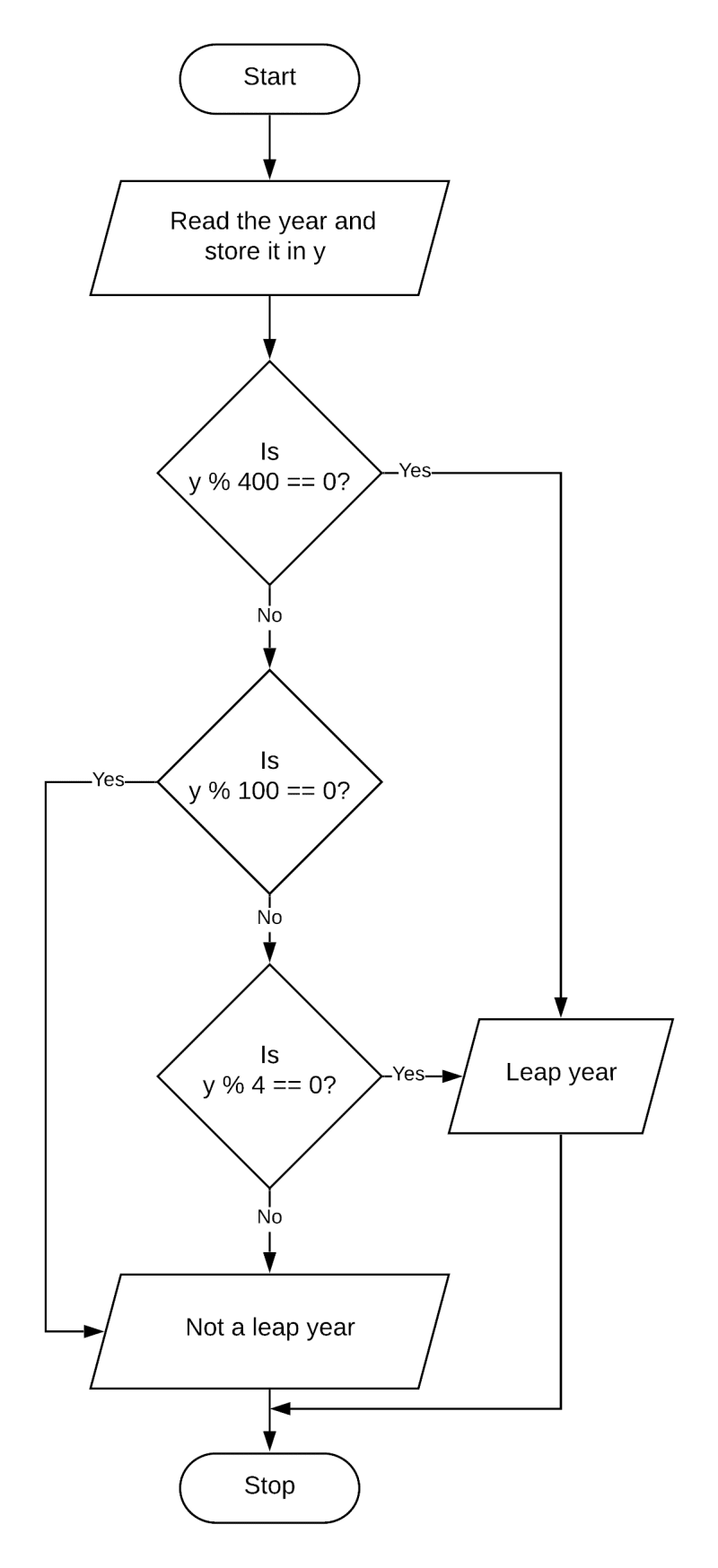# Algorithms and Flowcharts

## Fill in the blanks

#### Question 1

The step-by-step procedure to solve any problem is called Algorithm.

#### Question 2

Flowcharts are the pictorial representation of a program.

#### Question 3

The process of drawing a flowchart for an algorithm is known as Flowcharting.

#### Question 4

An algorithm should involve Finite number of steps to reach a solution.

#### Question 5

The Decision box is used for checking or applying any condition in the program.

## State True or False

#### Question 1

A flowchart is not a pictorial representation of steps to get the solution of a problem.
False

#### Question 2

Algorithm means a set of rules which specify how to solve a specific problem.
True

#### Question 3

Flowcharts are helpful in analyzing the logic of problems.
True

#### Question 4

Connectors are used to connect the boxes.
False

#### Question 5

The general direction of flow in any flowchart is from bottom to top or from right to left.
True

## Multiple Choice Questions

#### Question 1

In a flowchart, .......... indicate the sequence of steps and the direction of flow.

1. Flow lines ✓
2. Decision box
3. Processing box

#### Question 2

.......... is used to accept input and give output of a program.

1. Flow lines
2. Input/Output box ✓
3. Decision box

#### Question 3

The .......... represents the starting or ending point of a program.

1. Decision box
2. Flow lines
3. Start /Stop box ✓

#### Question 4

.......... connectors are used to join the parts of a flowchart contained within the same page.

1. On Page ✓
2. Off Page
3. None of these

## Draw the flowchart symbols for the following

#### Start:#### Stop:#### Input A, B:#### A= A + 1:#### Is A > B:#### Print A:#### Question 1

What is an algorithm? Write any three characteristics of an algorithm.

The step-by-step procedure to solve any logical and mathematical problem is called an Algorithm. Three characteristics of an algorithm are:

1. Input — An algorithm accepts an input.
2. Generality — An algorithm works in a set of inputs.
3. Definiteness — Each instruction should be written in a simple and precise manner so that everyone can understand it.

#### Question 2

Define the term flowchart. Write any two advantages of a flowchart.

A flowchart is a pictorial representation of the steps or an algorithm used to solve a particular problem. Two advantages of a flowchart are:

1. Communication — The pictorial representation of the flowchart provides better communication. It is easier for the programmer to explain the logic of a program.
2. Effective Analysis — It is a very useful technique, as flowchart is a pictorial representation that helps the programmer to analyze the problem in detail.

#### Question 3

What is the use of input/output box?

It is used for accepting inputs or giving output of the program.

#### Question 4

When do we use the Process box?

It is used for writing the processing instructions and doing calculations.

#### Question 5

What is the use of Decision box?

It is used for checking or applying any condition in the program.

## Write algorithms for the following problems

#### Question 1

Algorithm

Step 1: Start
Step 2: Open the shoe polish
Step 3: Put shoe polish on brush
Step 4: Polish one shoe
Step 5: Put shoe polish on brush
Step 6: Polish the other shoe
Step 7: Close the shoe polish
Step 8: Stop

#### Question 2

To input three sides of a triangle and print if it is scalene, isosceles or equilateral.

Algorithm

Step 1: Start
Step 2: Read three sides of triangle and store them in a, b, c.
Step 3: Check if a == b and b == c
Step 4: If true, print "Equilateral Triangle" and goto step 8
Step 5: Check if a == b or b == c or c == a
Step 6: If true, print "Isosceles Triangle" and goto step 8
Step 7: Print "Scalene Triangle"
Step 8: Stop

## Draw the following flowcharts

#### Question 1

Draw a flowchart in Microsoft Word for buying stationery from the market.

Flowchart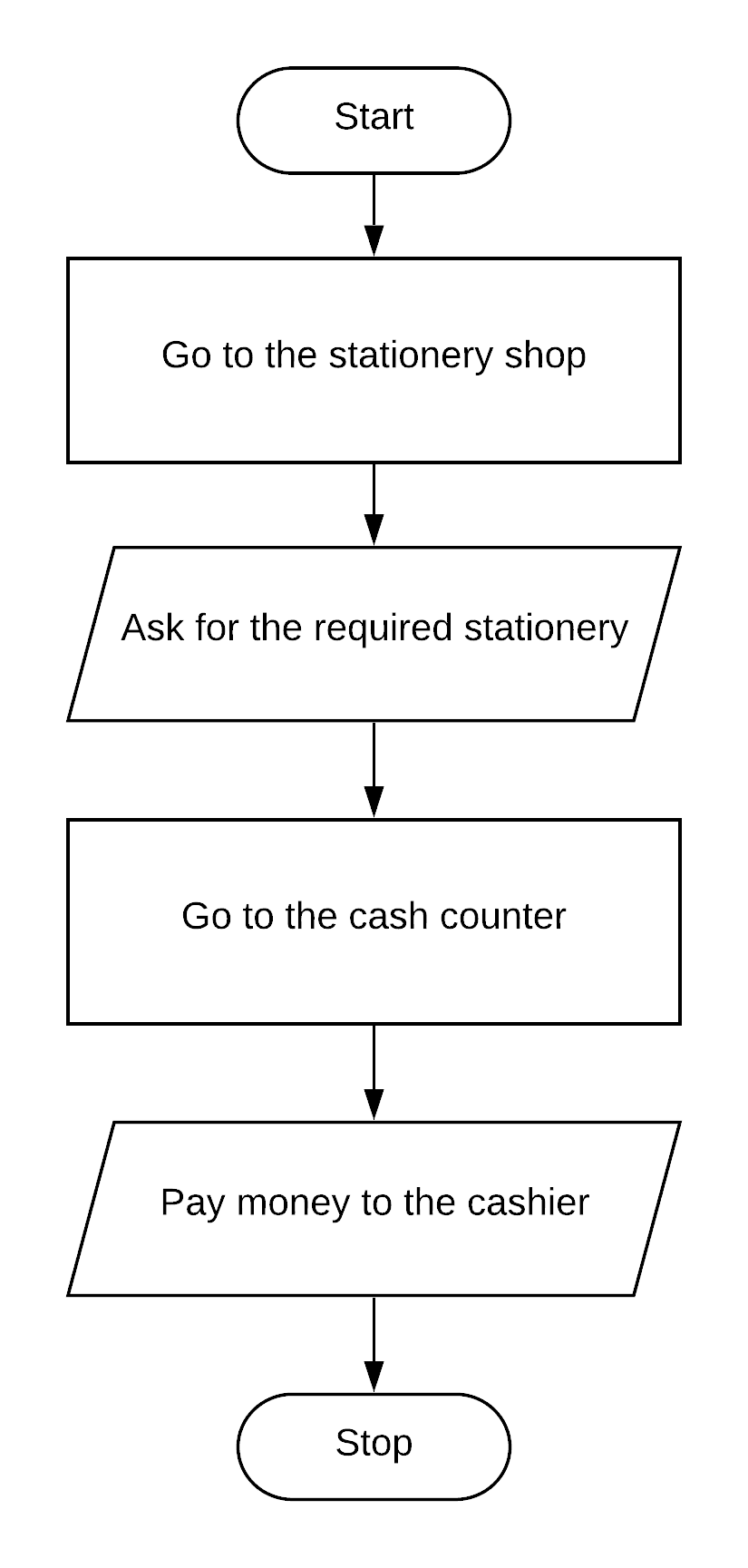#### Question 2

Draw a flowchart in Microsoft Word for the steps required to play music stored in a CD.

Flowchart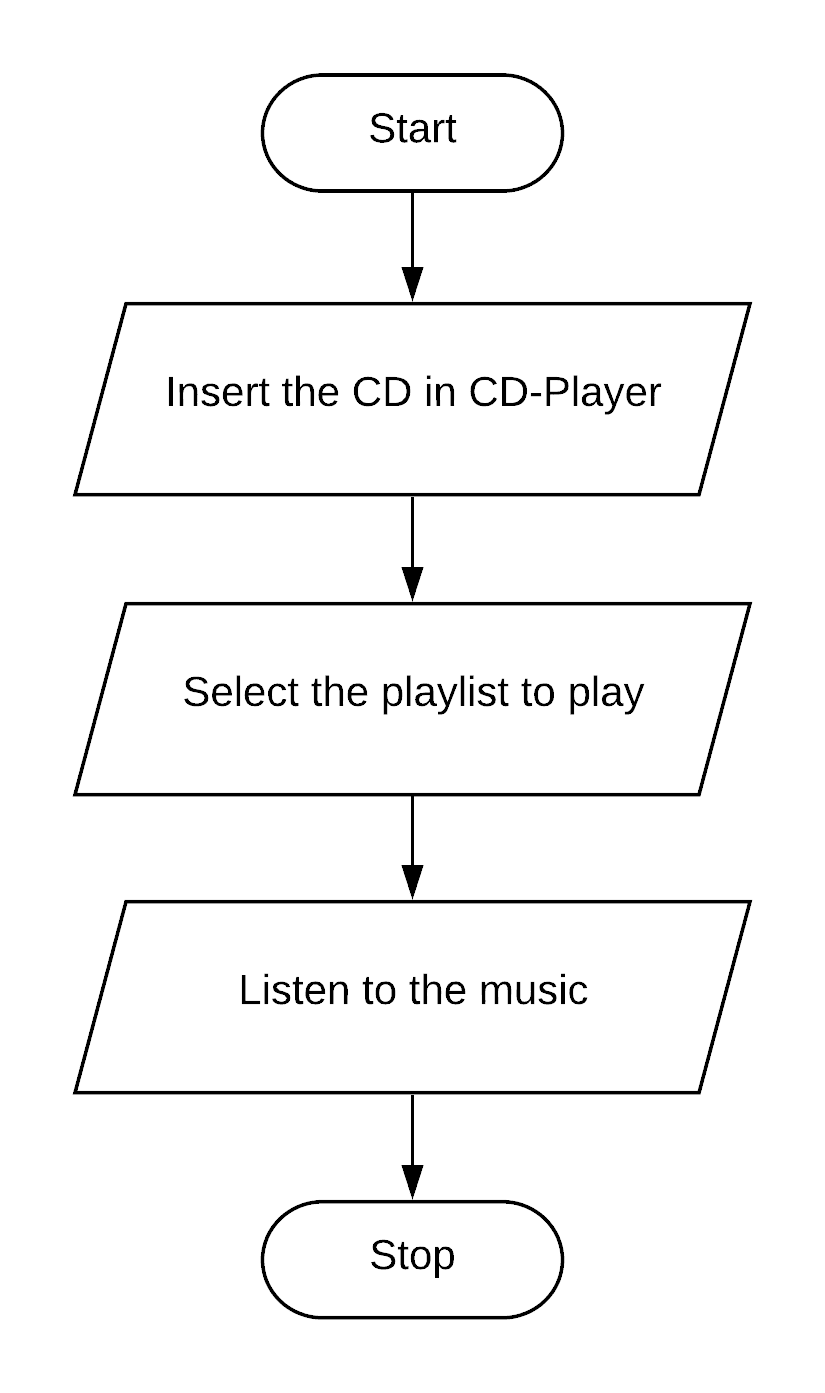#### Question 3

Draw a flowchart in Microsoft Word to accept two numbers, if the first number is greater than the second number, print their sum, otherwise print their product.

Flowchart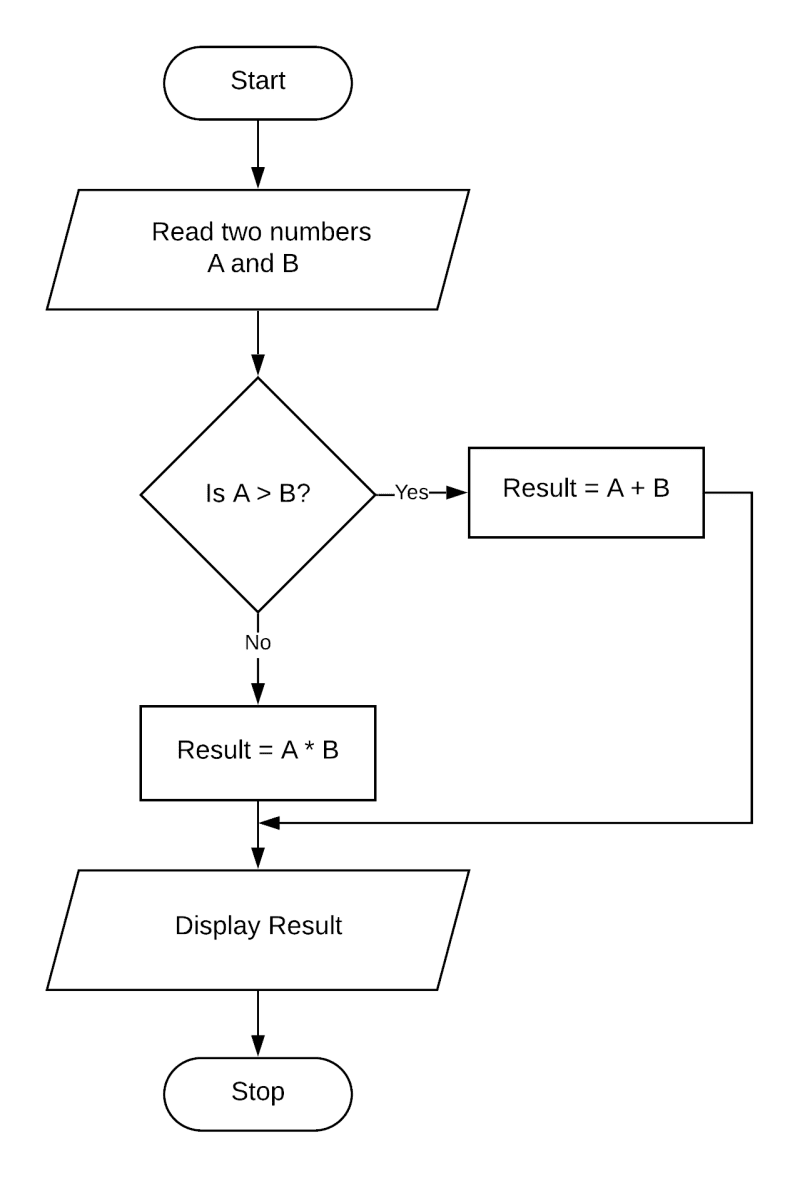#### Question 4

Draw a flowchart in Microsoft Word for the steps required to find the cost of 24 pens when the cost of one pen is Rs. 12.

Flowchart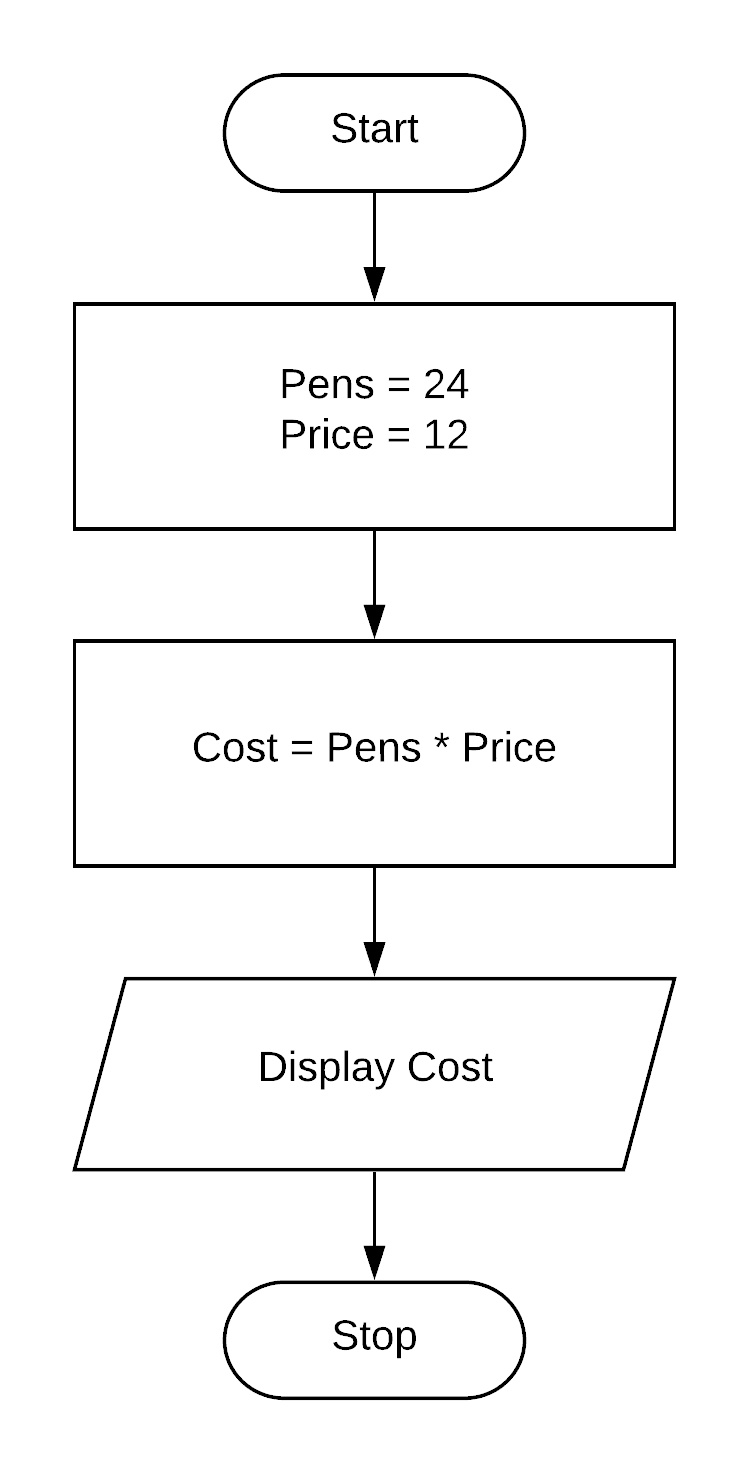## Write an algorithm and draw the flowchart for the following

#### Question 1

Finding the cube of a given number.

Algorithm

Step 1: Start
Step 2: Read the number and store it in a.
Step 3: Calculate cube of number as a * a * a
Step 4: Print cube of number
Step 5: Stop

Flowchart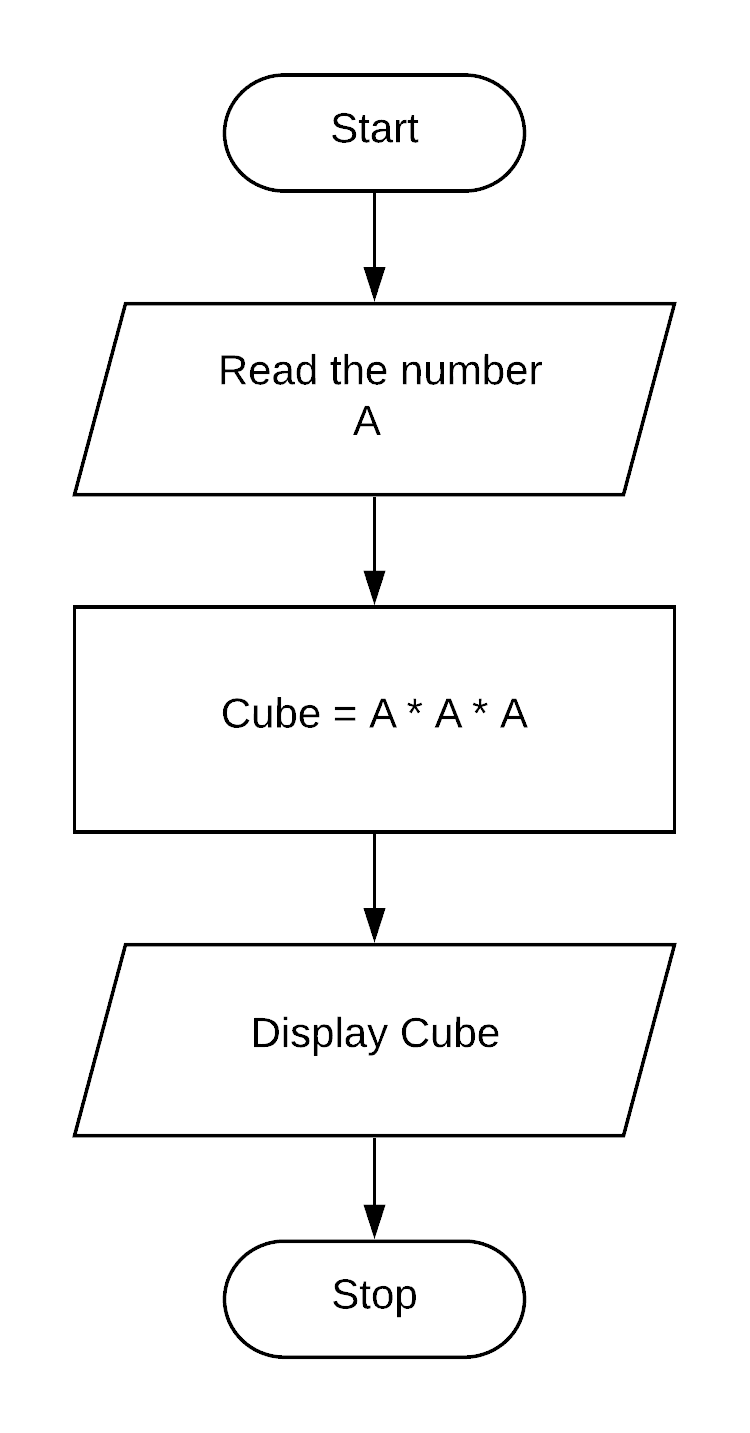#### Question 2

Print the smallest of two numbers.

Algorithm

Step 1: Start
Step 2: Read the two numbers and store them in a and b.
Step 3: Check if a < b
Step 4: If true then print a and goto step 6
Step 5: If false then print b
Step 6: Stop

Flowchart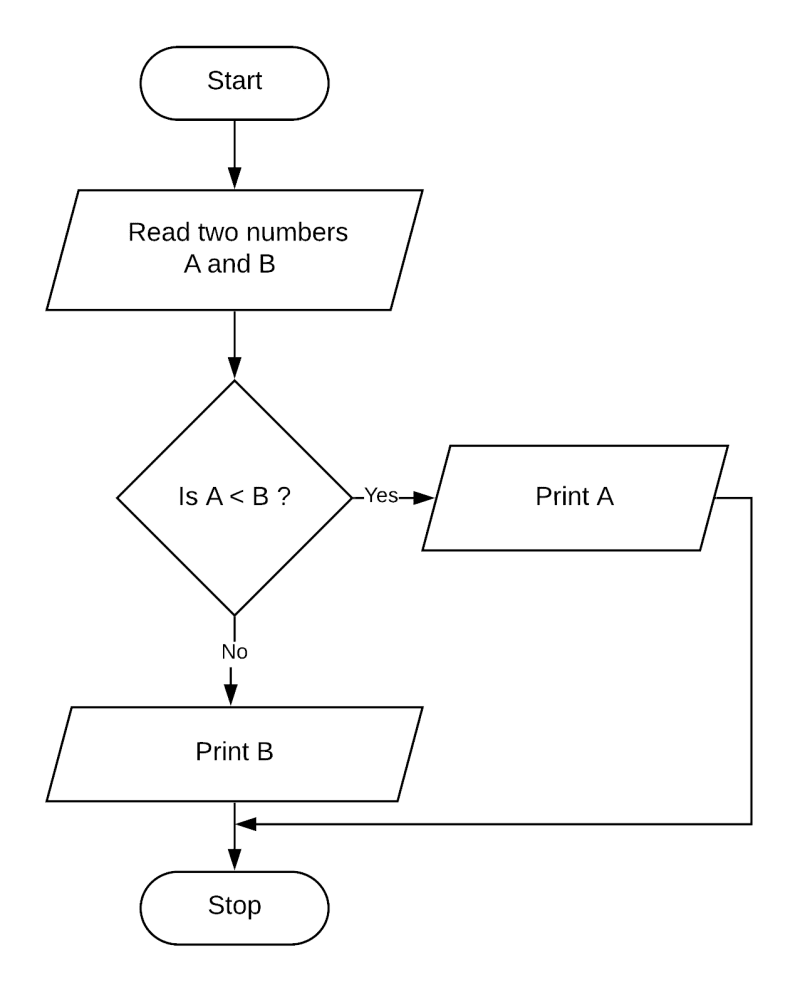## Correct the Flowchart symbols

#### Question 1

To wash the face

Corrected Flowchart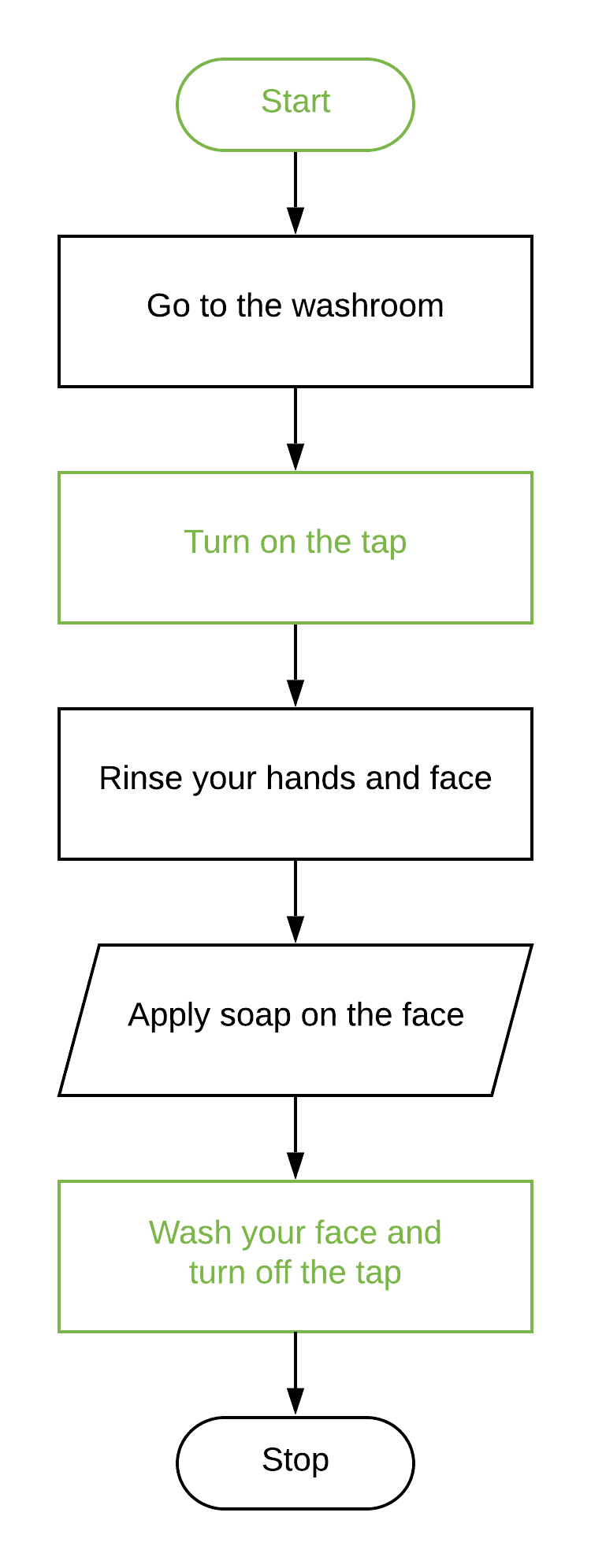#### Question 2

To multiply the two numbers

Corrected Flowchart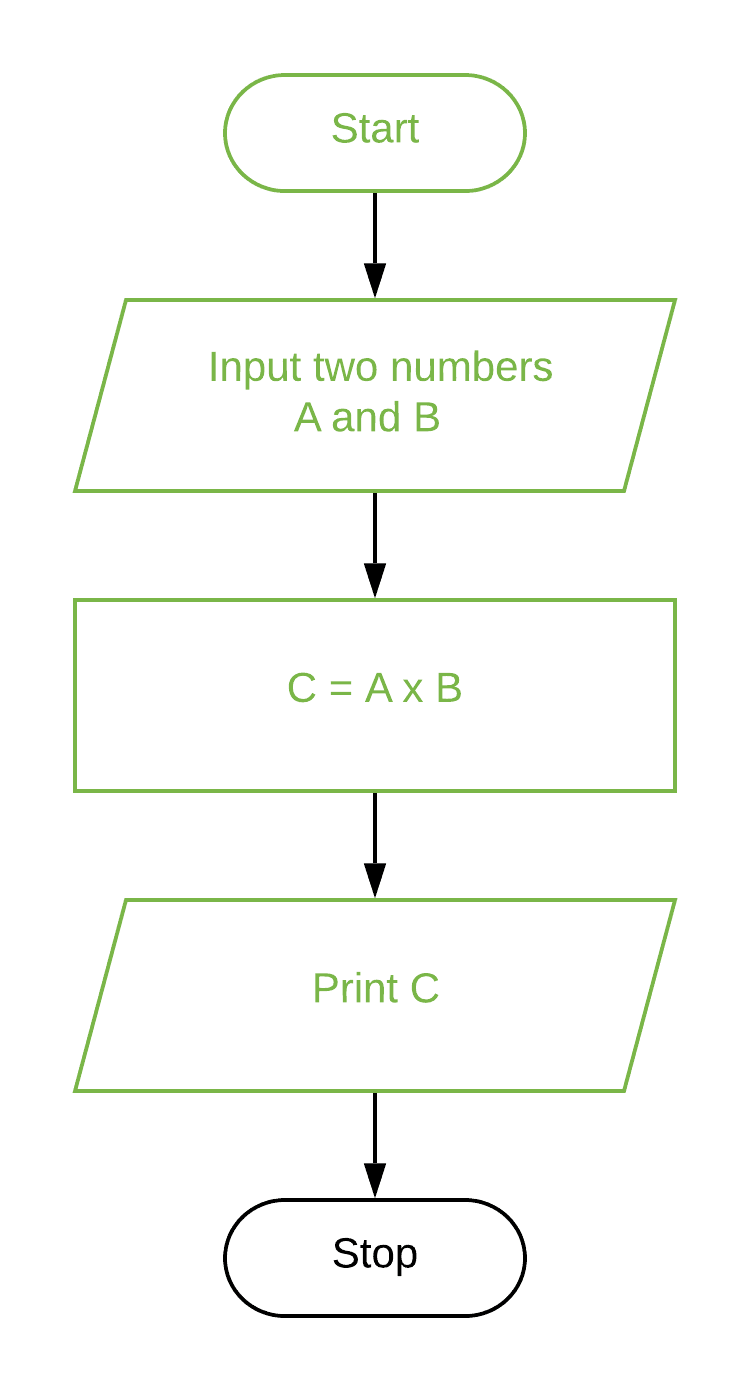## Make flowcharts for the following statements

#### Question 1

Accept the age of a person. Display the message Eligible For Role if the age is equal to or greater than 18, otherwise display the message Not Eligible.

Flowchart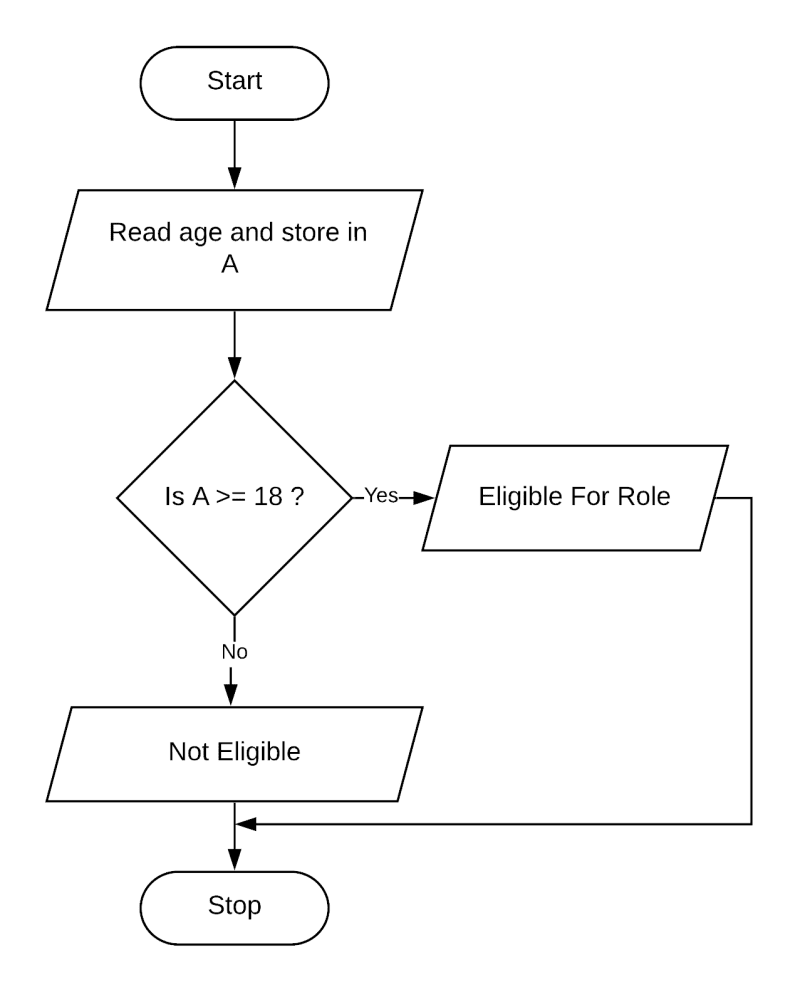#### Question 2

Input a name. If name is Kabir, then accept marks. If marks are >= 85, then display Good Performance else display Pass.

Flowchart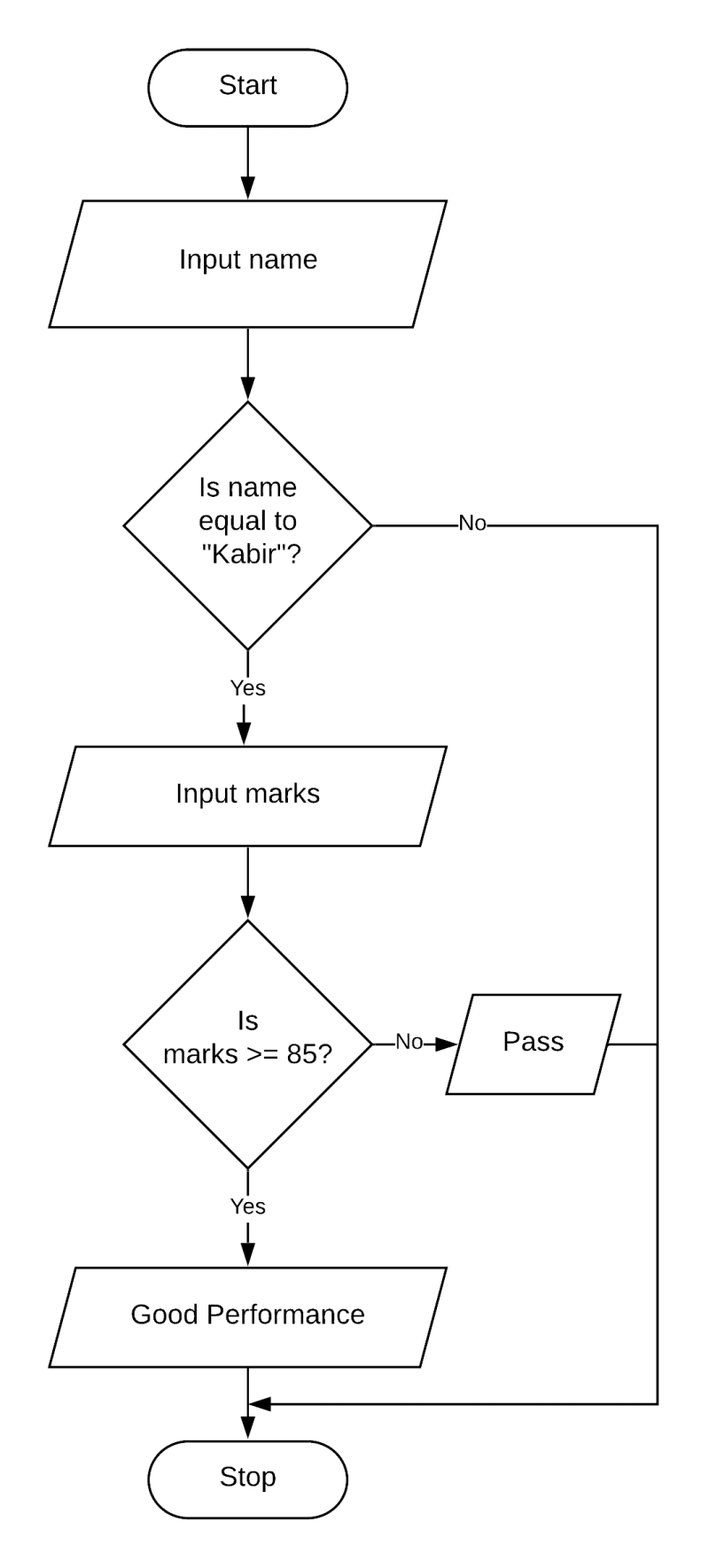#### Question 3

Accept one character. Display the message Vowel if the entered character is a vowel, otherwise display the message Not a Vowel.

Flowchart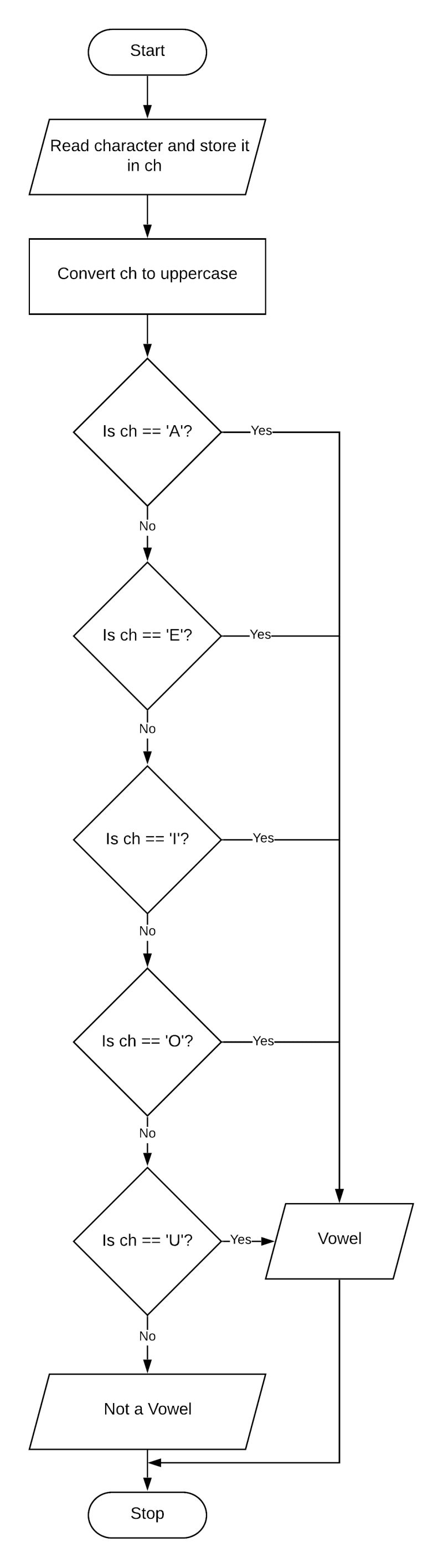#### Question 4

Input a year. Display the message Leap year if the entered year has 366 days, otherwise display the message Not a leap year.

Flowchart